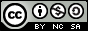- 目录 -
LeetCode 542 01 矩阵(BFS)

0 0 0
0 1 0
0 0 0


0 0 0
0 1 0
0 0 0


0 0 0
0 1 0
1 1 1


0 0 0
0 1 0
1 2 1


1. 给定矩阵的元素个数不超过 10000。
2. 给定矩阵中至少有一个元素是 0。
3. 矩阵中的元素只在四个方向上相邻: 上、下、左、右。

## 代码

  1 2 3 4 5 6 7 8 9 10 11 12 13 14 15 16 17 18 19 20 21 22 23 24 25 26 27 28 29 30 31 32 33 34 35 36 37 38 39 40 41 42 43  class Solution { public: struct node { int x, y, step; }; int go = {1, 0, -1, 0, 0, 1, 0, -1}; vector> updateMatrix(vector> &matrix) { int n = matrix.size(), m = matrix.size(); vector> result(n, vector(m, 99999)); vector> vis(n + 1, vector(m + 1, 0)); queue q; for (int i = 0; i < n; i++) for (int j = 0; j < m; j++) if (matrix[i][j] == 0) { q.push({i, j, 0}); vis[i][j] = 1; } while (!q.empty()) { node now = q.front(); q.pop(); result[now.x][now.y] = min(result[now.x][now.y], now.step); for (int i = 0; i < 4; i++) { int xx = now.x + go[i]; int yy = now.y + go[i]; if (xx >= 0 && xx < n && yy >= 0 && yy < m && !vis[xx][yy]) { if (matrix[xx][yy] == 1) q.push({xx, yy, now.step + 1}); else q.push({xx, yy, now.step}); vis[xx][yy] = 1; } } } return result; } };